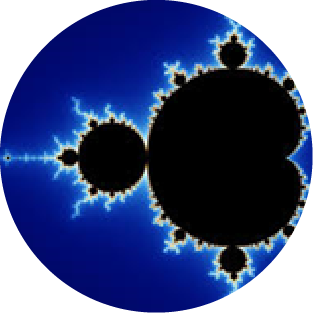## Banking and FinanceHave you ever wondered how a bank actually performs the calculation of your savings account interest, or the interest on your home mortgage loan?   How precise does your bank perform these calculations?  Are the round off errors contributing a significant amount of error?

Turns out that over time, small errors in the calculation of the agreed upon interest rates using a binary number system are worry-some enough to insist that banking calculations be performed in the decimal number system.  But why?  For one, you as a human typically negotiated an interest rate using the decimal number system, not the binary number system!  Therefore these interest amounts are decimal quantities and cannot be represented exactly in the binary number system.  For example, the interest rate of 3.15% cannot be exactly described by a binary number of any precision.

Further consider that many banking calculations are compounding.  For example, consider your credit card account.  The interest due is compounded on a daily basis, and you as a consumer end up paying interest on the interest!  Compounding financial calculations carry error from one calculation to the next so that the “real” value you owe, and the calculated value can and does differ; sometimes significantly.

Therefore, it is important to the financial industry that technologies and algorithms be available to support requirements for precise decimal calculation.  At MaiTRIX, we have known for some time that a residue based fractional value can support decimal fractions exactly.  In fact, our RNS-TPU is presently configured with a fractional range consisting of nearly 10 digits of “decimal like” fractional precision.  This provides our TPU unprecedented capability at processing decimal arithmetic at speeds and precision not available in the prior art.

Modular computation can perform banking and financial arithmetic in a precise and efficient manner, including market analysis and standard banking calculation.  At MaiTRIX we’re paying close attention to the many new and existing applications that benefit from the adoption of computers using unconventional arithmetic, and we see financial applications as a major market; we’re banking on it!

## Explore Modular ComputationHardware Matrix MultiplicationBanking and Financial ProcessingGeneral Purpose ComputationModular CircuitsDigital Signal ProcessingCryptography and SecurityMultiplier EfficiencyComputational MathematicsQuantum ComputingArtificial Intelligence3D IC technologySimulation and PhysicsFault Tolerance